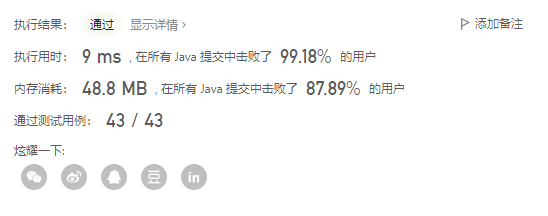> 文档中心 > LeetCode 每日一题——面试题 17.11. 单词距离

# 2.解题思路与代码

## 2.1 解题思路

1. 初始化 first 和 second 指向 0 位置2. first 先移动开始寻找 word1，找到第一个word1 后停止3. second 开始寻找 word2，找到第一个 word2 后停止，此时计算 first 和 second 之间的距离为 14. 此时first在前面，于是 first 继续寻找下一个 word1，寻找到后停止。此时计算 first 和 second 之间的距离为 25. 此时 second 在前面，于是开始移动 second 寻找下一个 word2，直到遍历完数组也没找到，遍历结束，返回最小值 1## 2.2 代码

``class Solution {    public int findClosest(String[] words, String word1, String word2) { int first = 0; int second = 0; int min = Integer.MAX_VALUE; boolean firstGo = true; while (first < words.length && second < words.length) {     while (firstGo) {  if(first >= words.length){      return min;  }  if (word1.equals(words[first])) {      firstGo = false;  } else {      first++;  }     }     while (!firstGo ) {  if (second >= words.length){      return min;  }  if (word2.equals(words[second])) {      firstGo = true;  } else {      second++;  }     }     min = Math.min(min, Math.abs(first - second));     if (first < second) {  first++;     } else {  second++;     } } return min;    }}``

## 2.3 测试结果# 3.总结

• 这道题使用双指针寻找两个字符串
• 当找到两个字符串后，计算距离，然后开始下一轮寻找
• 下一轮开始时，只移动位于前面的指针，后面的指针不动，即用前面的指针去寻找字符串